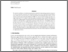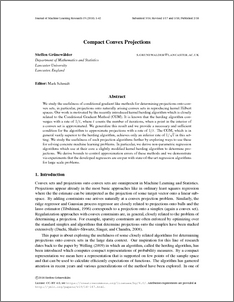# Compact Convex Projections

Grunewalder, Steffen (2018) Compact Convex Projections. Journal of Machine Learning Research, 18 (219). pp. 1-43. ISSN 1532-4435Preview
PDF (16-147)
16_147.pdf - Accepted Version

Text (16-147)
16_147.pdf - Published Version

## Abstract

We study the usefulness of conditional gradient like methods for determining projections onto convex sets, in particular, projections onto naturally arising convex sets in reproducing kernel Hilbert spaces. Our work is motivated by the recently introduced kernel herding algorithm which is closely related to the Conditional Gradient Method (CGM). It is known that the herding algorithm converges with a rate of 1=t, where t counts the number of iterations, when a point in the interior of a convex set is approximated. We generalize this result and we provide a necessary and sufficient condition for the algorithm to approximate projections with a rate of 1=t. The CGM, which is in general vastly superior to the herding algorithm, achieves only an inferior rate of 1=pt in this setting. We study the usefulness of such projection algorithms further by exploring ways to use these for solving concrete machine learning problems. In particular, we derive non-parametric regression algorithms which use at their core a slightly modified kernel herding algorithm to determine projections. We derive bounds to control approximation errors of these methods and we demonstrate via experiments that the developed regressors are en-par with state-of-the-art regression algorithms for large scale problems.

Item Type:
Journal Article
Journal or Publication Title:
Journal of Machine Learning Research
Uncontrolled Keywords:
/dk/atira/pure/subjectarea/asjc/2200/2207
Subjects:
Departments:
ID Code:
126822
Deposited By:
Deposited On:
10 Aug 2018 10:36
Refereed?:
Yes
Published?:
Published